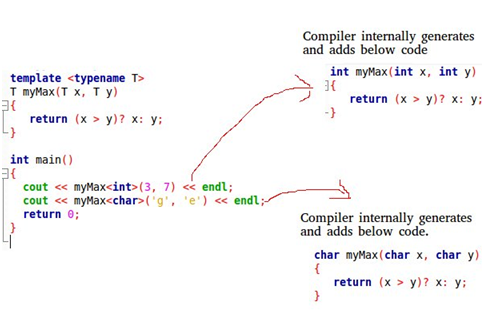# Templates in C++

Templates are considered simple yet powerful tools in C++. They work on a simple idea of passing a data type as a parameter. This helps programmers from writing the same code of different data types. For example, a renowned software company may contract you to create sort () for various data types. Instead of writing and maintaining multiple codes, you can write one sort () and pass data types as a parameter.

To support templates, C++ has two new keywords, namely ‘template’ and ‘typename.’ The keyword class can always be used to replace the second keyword – ‘typename.’

#### So how do templates work?

Like in macros, templates in C++ are expanded at compiler time. The only difference is that in C++, the compiler does type checking before performing template expansion. The idea here is the source code has only the function/class, while the compiled code may have several copies of the same function/class. The diagram below explains it better:#### Function Templates

These are generic functions that can be used for different data types. Common examples of function templates are max(), min(), sort(), and printArray()

#### How to declare a function template

To declare a function template, start with the keyword template followed by the template parameter. The parameter should be inside the <>. This is then followed by the function declaration. Check out the example below:
Template < class T>

T ourFunction (T arg)

{

. . . .. . . . . . .

}

Example 1

Write a C++ program that implements the bubble sort using templates.

// CPP code that implements bubble sort using the template function

#include

Using namespace std;

//a template function that implement bubble sort

//it can be used for any data type that supports comparison operator < and swap works for it

Template

Void bubble sort (T a[], int n) {

for (int k = 0; k < n-1; k ++)

for ( int f = n-1; i

if (a[ f ] < a [f-1])

swap (a [f], a[f-1]);

}

//Driver Code

Int main ( ) {

Int a  = {10, 30, 50, 40, 60, 20}

//This will call the template function

bubbleSort ( a,6);

cout<< “The sorted array is : “;

for ( int k = 0; k < n; k++)

cout<< a[k] << “ “;

cout<< end!;

return 0;

}

Running the programming above produces this output

The sorted array is : 10 20 30 40 50 60
Class Templates

Class templates are used when a class defines something independent of the data type. It can be used for classes such as BinaryTree, Stack, LinkedLists, Array, Queue, etc.

#### How to declare class template

Template

Class ClassName

{

. . . . . . . . .. . . . .

Public

T var;

T someOperation (T arg);

. .. . . . . .. . . .

};

Where

T – template argument which is a placeholder for the datatype used
Var and some Operation are both of type T
Get our C++ programming assignment help if you are struggling with assignments related to class templates.

Can a programmer specify the default value for template arguments?
Just like in normal parameters, you can specify default arguments to templates. The code below demonstrate how this can be done.

#include

Using namespace std;

Template < class T, class U = char >

Class A {

Public

T X;

U Y;

A() {

Cout<< “The constructor has been called” << end1; }

};

Int main () {

A a; //This command calls A

return 0;

}

The constructor has been called
The output produced is: